## Introduction

The optical Kerr effect is present in most materials but only becomes significant with very intense optical fields and/or long interaction times. In the limit of extreme nonlinearity, individual photons could be persuaded to interact strongly with one another and induce cross-phase modulation (XPM). This kind of interaction is a basis of the deterministic control-not gate and phase-not gates that lie at the heart of quantum computing algorithms.1,2 In addition, a large XPM can also be used for generation of cluster states, which are the basis for one-way quantum computing,3,4,5 as well as for implementation of nonlinear optical switches.6

To date, there have been various proposals aimed at realizing this strong interaction. Optical fibers are an attractive XPM medium.7 While they may not be highly nonlinear, the interaction times can be extended simply by using longer fibers. Unfortunately, the fast response time of optical fibers makes it impossible to temporally mode-match two copropagating single photons.8 Even if the nonlinearity is large, the fast response of the medium implies that only a small and randomly distributed portion of the single-photon wave packet experiences the phase shift.9 In the slow response regime, on the other hand, noise sources are always required to preserve the input/output canonical relations in nonlinear processes.9,10,11

Another method of facilitating long interaction times for XPM is via light interaction with an atomic ensemble. Electromagnetically induced transparency (EIT)12 based XPM exploits slow-light effects in an atomic ensemble to enhance the nonlinear interaction of light fields via the ac-Stark effect.13 It was shown that a phase shift (10−5 rad) almost two orders of magnitude larger than that in optical fibers can be achieved using the EIT system.14,15 It has, however, been theoretically demonstrated that EIT-based XPM suffers from severe loss in regimes where large phase shifts are expected. Gea-Banacloche16 showed that spontaneous emission into the initially unoccupied temporal modes is responsible for the small XPM in an EIT medium. In this scenario, a large phase shift is only possible in the limit in which the pulse bandwidth matches the medium bandwidth.16 The EIT medium in this regime, however, becomes ineffective and noisy. This is because some frequency components of one light field, lying outside the EIT window, can lead to spontaneous emission that is ultimately responsible for weak and low fidelity XPM. Moreover, the storage efficiency limit of 50%17 in EIT-based systems poses a practical limit on the fidelity of the output states.

In this paper, we propose a scheme based on storing two light fields via a Raman process in a Λ gradient echo memory (Λ-GEM).18 The simultaneous storage of the interacting light fields allows for greater interaction time than schemes where one field freely propagates through the memory medium.19 The nature of quantum memory in GEM is such that it stores different frequency components (modes) of the initial light pulse at different spatial locations. This property of the memory ensures that different modes at different spatial locations do not interact with one another. Thus, one can avoid the cross-mode spontaneous noise in XPM and multimode complications affecting the EIT-based systems outlined recently in Ref. 16. The medium bandwidth can be tuned to match the bandwidth of the probe field without otherwise changing the inherent nonlinearity of the system. This is because, all frequency components inside the broadened Raman profile experience the same absorption and therefor the loss due to the spontaneous emission for those frequencies will be negligible. We will start our discussion with an outline of the scheme and present results of our theoretical modeling. We then present a proof of principle experiment that shows XPM in a Λ-GEM system.

## Materials and methods

In Λ-GEM, a weak optical field is Raman coupled into the spin coherence of an ensemble of three-level atoms using a strong coupling field.18 A linearly varying ground-state splitting is applied using a magnetic field gradient, η, such that the light pulse is decomposed into its constituent Fourier components that are then stored longitudinally along the storage medium. Reversing the sign of the gradient will time-reverse the absorption leading to a coherent photon echo. This storage scheme has been proven to be versatile, efficient and noiseless.20,21,22

To understand the dynamics in GEM, we use a polariton that is a superposition of the electric field,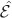, and atomic spin coherence,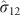, in the spatial Fourier domain,23 defined as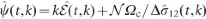, where k is the spatial frequency,is the effective linear atomic density, Δ is the Raman detuning from the excited state, and Ωc is the coupling field Rabi frequency. During storage, the polariton evolves to higher k-values at a rate proportional to η. When using GEM for XPM, it is the polariton that will be phase shifted, leading to a phase shift of the photon echo on recall from the memory.

There are three properties of the polariton that are important to the following discussion:

1. i)

The Fourier transform of the Maxwell equation gives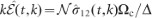.23 Because the spin coherence has a constant amplitude during storage, the Maxwell equation implies that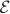is inversely proportional to k.

2. ii)

The polariton can be stopped in k-space by switching η to 0. For a pulse stored with η=0, the group velocity of the optical component is found to be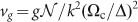.

3. iii)

The polariton is purely atomic,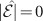, when the coupling field is off.

We will now analyze a scheme in which the probe and signal fields are simultaneously stored in a double-GEM system. Since the origin of this XPM is the ac-Stark effect, the effective phase shift can be enhanced by increasing the interaction time.

The Rb level structure and nonlinear interaction scheme between two photons stored inside the memory are shown in Figure 1a. The two fields (with Rabi frequencies of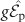and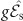) are stored independently in two atomic spin coherences,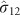and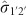, using two coupling fields with Rabi frequencies of Ωc and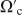.

The probe and signal enter the medium consecutively. By timing the two coupling fields, each pulse is independently mapped into distinct polaritons. These modes propagate in opposite directions in k-space due to the opposite sign of the hyperfine Zeeman sublevels. The k-space evolution of the two polaritons is stopped at a constant k by switching η to zero soon after the signal pulse enters the medium. The coupling field for the probe is then switched off, mapping the polariton into the spin coherence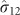. The coupling field for the signal is left on, ensuring a photonic component of this polariton that will give rise to an ac-Stark shift of the probe field polariton. The maximum available interaction time is proportional to the memory length and inversely to the group velocity of the signal light. After a controllable interaction time, the frequency gradient and coupling field Ωc can be switched to recall the probe, which will be phase shifted due to the interaction with the signal. The interaction Hamiltonian of the system with level scheme depicted in Figure 1a can be written as: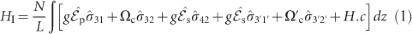where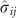are the collective atomic spin operators, g is the atom–field coupling constant, N is total number of atoms in the interaction volume and L is the ensemble length. The phase of the coherence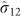after the interaction time is then given by: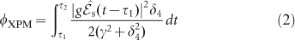and the loss rates of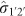and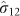are given by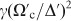and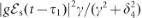, respectively.

## Results and discussion

We numerically solve the Maxwell–Bloch equations to semiclassically model the storage and nonlinear interactions between two coherent states with mean photon number of one. The evolution of the signal and probe polaritons is shown in Figure 1b. The XPM resulted from nonlinear interaction between the signal and probe polaritons occurs during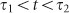, when the gradient field is switched off. We have observed a linear increase in the phase of the retrieved probe field as a function of the signal field intensity. The simulation show no change in the phase of the probe field as its intensity increased, showing immunity to self-phase modulation (SPM).11 The interaction strength is limited by the storage of the signal field which still has a non-zero group velocity in the memory. In order to increase the interaction strength and interaction time, one can use a pair of counter-propagating coupling fields to generate stationary light inside the memory. The application of a counter-propagating coupling field for the signal field would allow stationary trapping of the signal light. The physics of the trapping light in this case is similar to stationary light generated in an EIT medium that has previously been experimentally demonstrated.24 The stationary light in GEM is generated through an off-resonance interaction and therefore suffers less from loss compared to EIT. The phase shift on the probe field due to the signal field is shown as a function of interaction time between them in Figure 1c. Similar results have been obtained for the phase shift of a strong coherent state (α≈102) resulting from interaction with a signal pulse with mean photon number of one. Although the nonlinear phase shift for the proposed scheme is smaller than π, the current scheme can be used to implement parity or phase gates where the strength of the coherent states can offset the weakness of the nonlinearities.2,25 The simulation results suggest that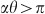is achievable in our scheme and therefore the error in discriminating the final states (even and odd parity states) can be less than 10−3,25,26 which is near-optimal.

We also perform quantum simulations by solving the master equation numerically. The interaction scheme in this case is simplified, so it can be solved using our available ultrafast computer. The quantum simulation, performed on the system during the interaction time (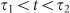), yields useful information regarding the noise and gate imperfections. For this type of simulation, it is assumed that initially a photon is encoded in a coherence between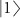and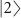so the initial state of the atomic system becomes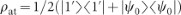, where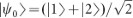. The initial state of the incoming signal photon is then given by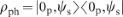, where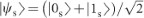, giving the total initial state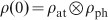. We also assume that the signal photon interaction is in the form of stationary light.

Next, we solve the master equation including Langevin noise terms. From the resulting density operator, the conditional phase shift between a single photonic qubit in state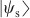and polaritonic qubit encoded in the atomic coherence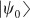is calculated as a function of interaction time. The conditional phase shift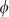and gate fidelity are shown in Figure 1d for parameters closely corresponding to the semiclassical simulations. The fidelity calculated here is that of a two-qubit controlled phase gate using single photons.27 This fidelity is low, as expected, since the interaction between single photons is very fragile. This is not such an issue for the parity gate described above since the interaction between a single photon and large coherent state is more robust against noise. Decoherence sources such as decay of the signal field amplitude due to Raman scattering (with lifetime of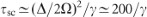), spontaneous emission decay and atomic spin dephasing are included in the model. We found that the noise due to spontaneous emission is the dominant source of fidelity degradation.

Now we present proof of principle experimental results for nonlinear interaction between a stored coherent state and a propagating one. Although this experiment does not implement the full seven-level scheme outlined above, the results support our theoretical models with respect to the XPM strength and SPM properties. The experimental setup is shown in Figure 2a. A coupling field and a weak probe field were combined using a ring resonator and sent into a 20-cm long gas cell of 87Rb mixed with 0.5 torr of Kr buffer gas. The gas cell was housed inside a pair of solenoids that generate switchable magnetic field gradients. After the memory, the coupling field was suppressed using a 85Rb gas cell and the probe beam was measured using heterodyne detection (see Ref. 22 for more experimental details).

The probe was stored for approximately 15 μs, while the coupling field was switched off. During that time, a signal field generated from a diode laser and detuned by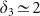GHz from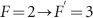of 87Rb D2 line was sent through the memory. This field was counter-propagating with respect to the probe and coupling fields to avoid measurement contamination. On recall, therefore, the stored probe field will be phase shifted proportional to the strength and duration of the signal field. To measure the size of the phase shift, we ran two storage experiments in succession, without and with the signal field, as shown in Figure 2b ((i) and (ii) respectively). A phase reference for the two recalled probe pulses was provided by a pulse that passed through the memory cell 10 μs before the start of each experiment. This reference pulse then allowed us to compare the recalled probe phase with and without the signal field, as indicated in the figure.
To verify that this phase shift is due to a nonlinearity in the memory, we measured the phase shift as a function of the signal field Rabi frequency, as shown in Figure 3a, where the solid line represents the theoretical expectation calculated19 using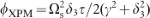, where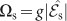is the signal field Rabi frequency and τ is its duration. As predicted by theory, our scheme has no measurable SPM, as shown in Figure 3b, where the recalled probe phase is seen to be independent of the probe intensity.